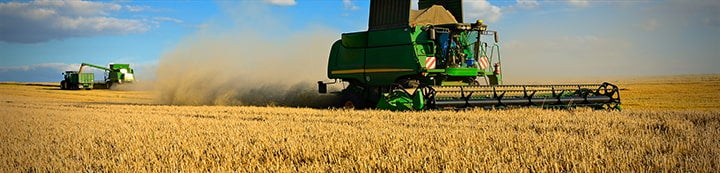1 - 6 of 6 Posts

jackaroo

·
Registered
Joined
·
188 Posts
Discussion Starter · ·
I can't seem to remember how to figure plants per sq foot on my 9 inch spacing! I'm sure someone with better memory than me could tell me????

BrianTee

·
Joined
·
5,855 Posts
144/9

·
Registered
Joined
·
1,912 Posts
12/9 = 1.33 foot of row = square foot.

jesseb99

·
Registered
Joined
·
401 Posts
There are 43560 square feet per acre if you take that number divided .75 (nine divided by 12 all this does is convert inches into feet) that should give you the number of linear feet there are in one acre of a nine inch row. It should figure out to be 58,080 feet of nine inch row would equal one acre. From here it is a simple calculation to figure how many seeds per foot by taking the total population divided by your feet of row. For example if you wanted 160,000 seeds per acre you would divide 160,000 by 58,080 to get 2.75 seeds per foot of nine inch row if my calculating is correct. I am not sure if this what you are asking though. If you just want seeds per square foot and not seeds per foot of row all you need to do is divide your total population by 43,560 and that should give you simply seeds per square foot not the number of seeds you want to count in one foot of your nine inch row.

cobra1970

·
Registered
Joined
·
293 Posts
I can't seem to remember how to figure plants per sq foot on my 9 inch spacing! I'm sure someone with better memory than me could tell me????

Count the plants in one row for a lineal foot. Take that number and multiply by 1.33 to get your plants/ft2 on a 9" spacing machine.

jackaroo

·
Registered
Joined
·
188 Posts
Discussion Starter · ·
Thanks. Just seeing how poor my stands are1 - 6 of 6 Posts